## Particle-Fluid Coupling Algorithm Considering Dynamic Fluid Mesh

HE Jinhui, LI Mingguang,, CHEN Jinjian, XIA Xiaohe

Department of Civil Engineering, Shanghai Jiao Tong University, Shanghai 200240, China

 基金资助: 国家自然科学基金面上项目(41977216)国家自然科学基金面上项目(51978399)上海市科委项目(BI0100054)Abstract

It is generally difficult to consider the fluid dynamic boundary problem in the traditional particle-fluid coupling algorithm, causing calculation errors owing to the mismatching of the fluid-solid boundary and affecting the accuracy of the results in the modeling of large deformation issues. In view of this problem, the dynamic updating method of fluid mesh is introduced and Darcy’s seepage equation and the particle-fluid interaction equation in dynamic mesh are derived. Based on the discrete element commercial software PFC2D, the particle-fluid coupling algorithm considering dynamic fluid mesh is developed. The proposed algorithm is applied to simulate the undrained shear biaxial test of saturated soil. The comparison result with the constant volume method verifies the effectiveness of the developed algorithm. Finally, the algorithm is used to simulate the undrained biaxial tests at different confining pressures. The law of the calculated results agrees well with that of the laboratory tests. By considering the problem of fluid dynamic boundary, the developed algorithm can obtain the fluid-solid boundary matching in the simulation of triaxial compression test and one-dimensional consolidation test or other cases in large deformations, which can help to improve the simulation accuracy and offer a theoretical reference for similar studies.

Keywords： fluid-solid coupling; dynamic mesh; constant volume method; biaxial test

HE Jinhui, LI Mingguang, CHEN Jinjian, XIA Xiaohe. Particle-Fluid Coupling Algorithm Considering Dynamic Fluid Mesh[J]. Journal of shanghai Jiaotong University, 2021, 55(6): 645-651 doi:10.16183/j.cnki.jsjtu.2020.317

## 1 颗粒-流体耦合算法原理及程序实现

### 1.1 颗粒场分析

$mpap=∑cfc+fstatic+fd$
$Ipαp=∑crc×fc+Md$

### 1.2 流体场分析

$uim=ui1+m-1a-1uia-ui1$
$xjn=xj1+n-1b-1xjb-xj1$

$v-=1N∑k=1Nvk$
$X-=1N∑k=1NXk$

$vrelk=vk-v-$
$Xrelk=Xk-X-$

$vprek=GXrelk$

$δ=∑k=1N(vprek-vrelk)2$

$∑p=1NGrsxrel,spxrel,tp=∑p=1Nvrel,rpxrel,tp$

$εv=ε·vΔt=12(Gww+G'ww)Δt=GwwΔt$

$Δp=Bfεv$

$vgh=2KKhK+Khp-phUg-Ughγw$

### 图1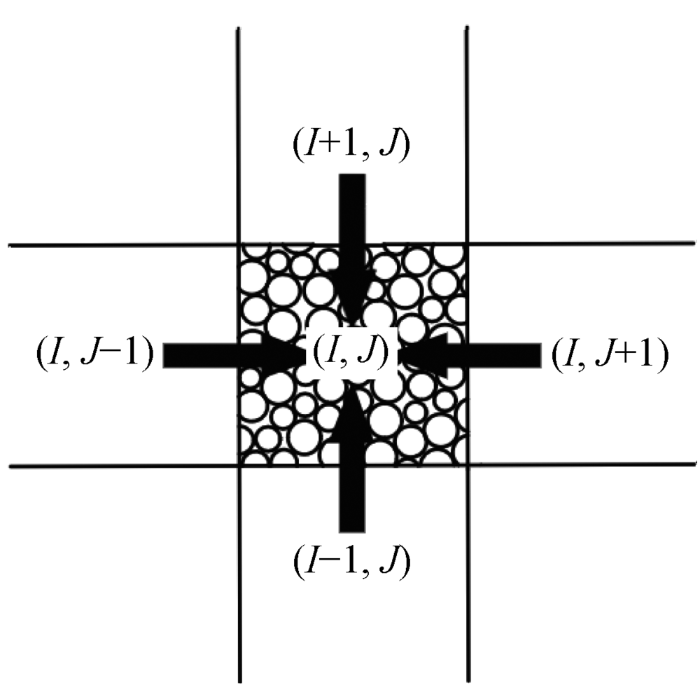Fig.1   Schematic diagram of seepage action between a certain mesh and adjacent meshes

$v'gh=vgh+ugp0$

$Q=∑g=12∑h=12v'ghSghΔt$

$p'darcy=BfQVcell$

$pnew=pold+Δp+p'darcy$

### 1.3 两相耦合场分析

$fstatic=pnewAcrn$

### 图2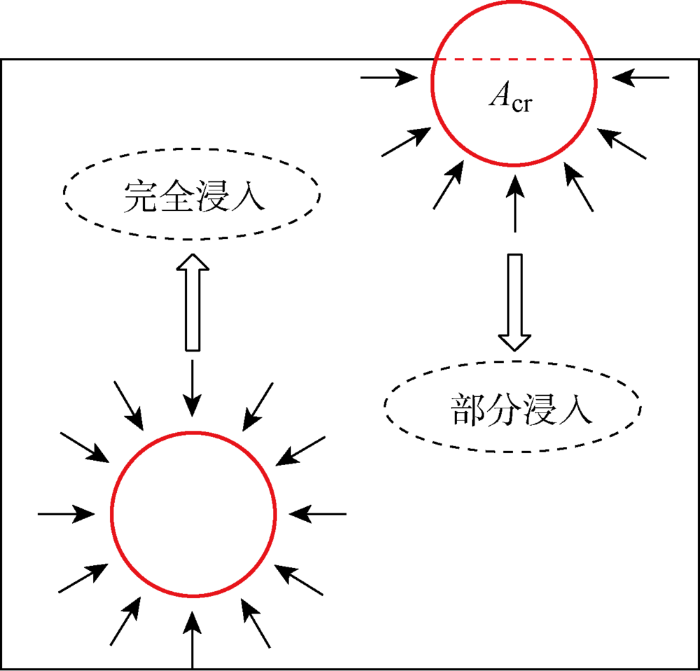Fig.2   Schematic diagram of positions of particles and their stress states in a fluid mesh

### 图3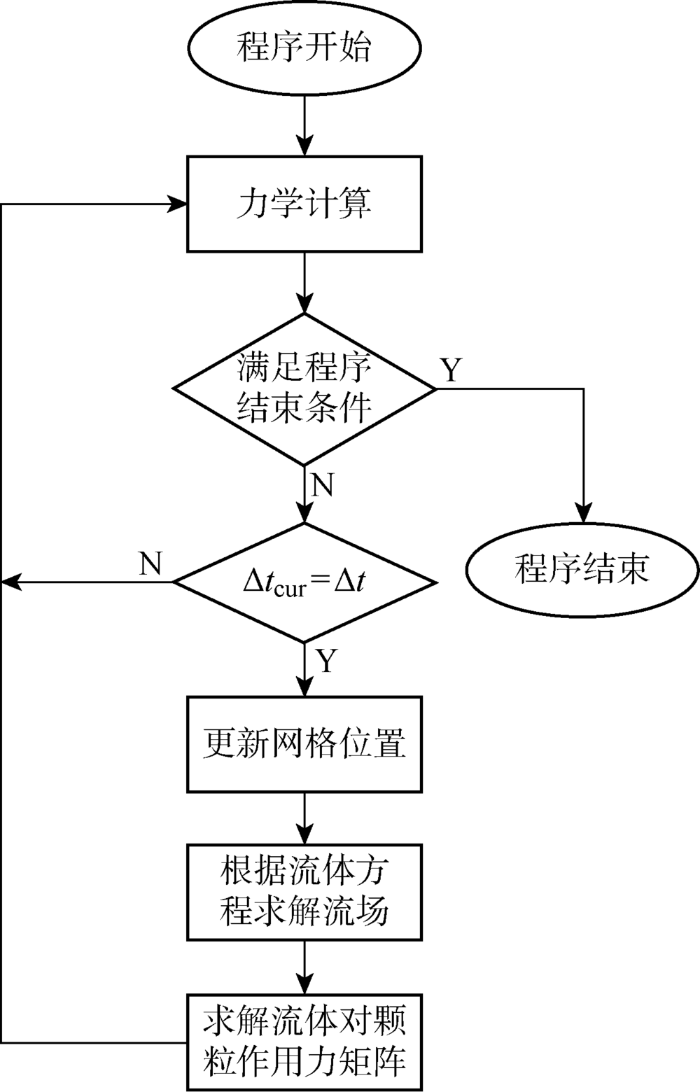Fig.3   Schematic diagram of coupling process

## 2 颗粒-流体耦合算法验证

### 图4Fig.4   Diagram of grain size distribution

Tab.1  Particle parameters

### 图5Fig.5   Schematic diagram of biaxial test model

### 2.2 常体积法原理

$εv=2vaΔτWx-2vrΔτWyWxWy=0$

$vr=WxWyva$

### 图6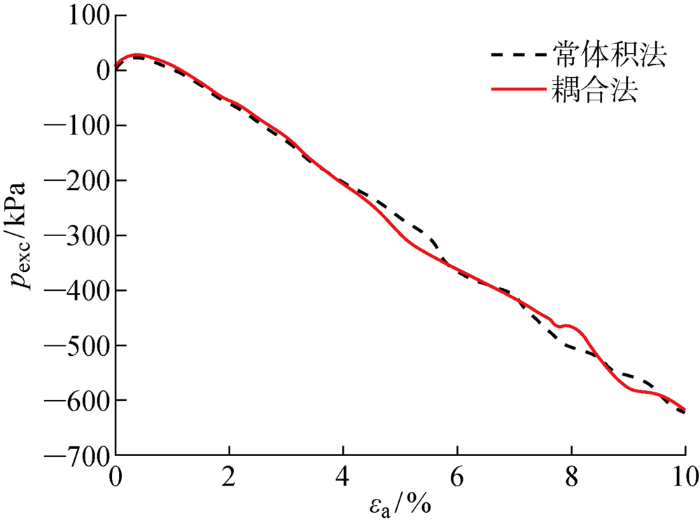Fig.6   Comparisons of excess pore water pressure in coupling method and constant volume method

### 图7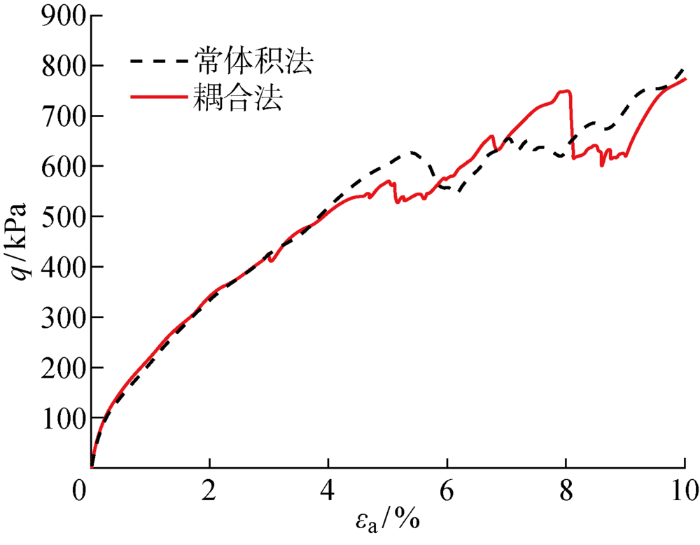Fig.7   Comparisons of deviatoric stress results in coupling method and constant volume method

## 3 不同围压影响下的双轴试验结果分析

### 图8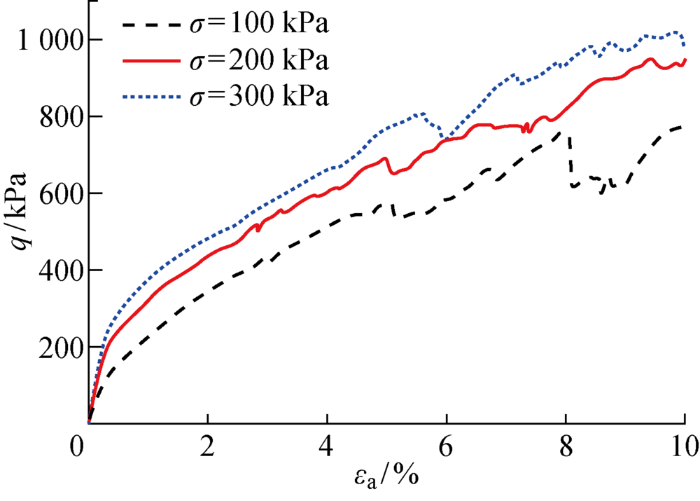Fig.8   Comparison of deviatoric stress results at different confining pressures

### 图9Fig.9   Comparisons of excess pore water pressure results at different confining pressures

### 图10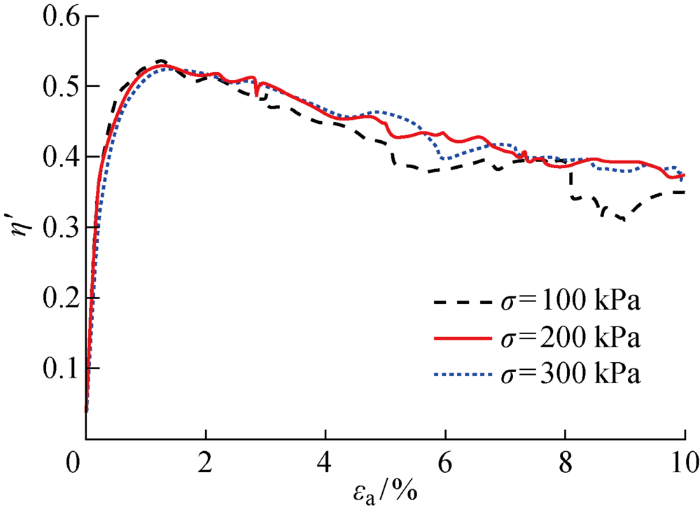Fig.10   Comparisons of effective stress ratio results at different confining pressures

## 4 结论

(1) 在引入流体网格动态更新方法的前提下,改进并推导动网格下的达西渗流方程和颗粒-流体相互作用方程,在离散元商业软件PFC2D基础上开发了考虑动态流体网格的颗粒-流体耦合模块.该算法原理简单,易于实现,弥补了传统微观耦合方法的不足,为大变形微观耦合模拟提供了参考,但在流场可视化、算法效率等方面仍需进一步的改进和研究;

(2) 将算法用于模拟饱和土不排水剪切双轴试验,并通过和常体积法的结果对比,验证了算法的有效性.结果显示,两种方法模拟得到的偏应力曲线和孔压曲线规律一致,数值较为接近.

## 参考文献 原文顺序 文献年度倒序 文中引用次数倒序 被引期刊影响因子

[J]. 岩土工程学报, 2014, 36(5):793-801.

JIANG Mingjing, ZHANG Wangcheng.

Coupled CFD-DEM method for soils incorporating equation of state for liquid

[J]. Chinese Journal of Geotechnical Engineering, 2014, 36(5):793-801.

[J]. 岩土力学, 2016, 37(1):210-218.

LIU Guang, RONG Guan, HOU Di, et al.

Fluid-particle coupled model and a numerical investigation on undrained shear behavior of saturated soil

[J]. Rock and Soil Mechanics, 2016, 37(1):210-218.

ZHANG Z L, SHAO Z S, FANG X B, et al.

Research on the fracture grouting mechanism and PFC numerical simulation in loess

[J]. Advances in Materials Science and Engineering, 2018, 2018:1-7.

ZENG Y W, XIA L.

Numerical simulation of hydraulic fracturing in transversely isotropic rock masses based on PFC-2D

[J]. Journal of Vibroengineering, 2019, 21(4):833-847.

WANG Y, DONG Q, CHEN Y.

Seepage simulation using pipe network flow model in a discrete element system

[J]. Computers and Geotechnics, 2017, 92:201-209.

[D]. 福州: 福州大学, 2011.

WANG Xuehong.

Discrete element modelling of mechanisms of high frequency hydraulic vibratory pile driving

[D]. Fuzhou: Fuzhou University, 2011.

ZEGHAL M, EL SHAMY U.

A continuum-discrete hydromechanical analysis of granular deposit liquefaction

[J]. International Journal for Numerical and Analytical Methods in Geomechanics, 2004, 28(14):1361-1383.

TAO H, TAO J.

Quantitative analysis of piping erosion micro-mechanisms with coupled CFD and DEM method

[J]. Acta Geotechnica, 2017, 12(3):573-592.

ZHANG A, JIANG M, THORNTON C.

A coupled CFD-DEM method with moving mesh for simulating undrained triaxial tests on granular soils

[J]. Granular Matter, 2020, 22(1):1-13.

ZHANG F, WANG T, LIU F, et al.

Modeling of fluid-particle interaction by coupling the discrete element method with a dynamic fluid mesh: Implications to suffusion in gap-graded soils

[J]. Computers and Geotechnics, 2020, 124:103617.

SHAFIPOUR R, SOROUSH A.

Fluid coupled-DEM modelling of undrained behavior of granular media

[J]. Computers and Geotechnics, 2008, 35(5):673-685.

LIU G, RONG G, PENG J, et al.

Numerical simulation on undrained triaxial behavior of saturated soil by a fluid coupled-DEM model

[J]. Engineering Geology, 2015, 193:256-266.

KHALILI Y, MAHBOUBI A.

Discrete simulation and micromechanical analysis of two-dimensional sa-turated granular media

[J]. Particuology, 2014, 15:138-150.

Coupling pore-water pre-ssure with distinct element method and steady state strengths in numerical triaxial compression tests under undrained conditions

[J]. Landslides, 2007, 4(4):357-369.

KEISHING J, HANLEY K J.

Improving constant-volume simulations of undrained behaviour in DEM

[J]. Acta Geotechnica, 2020, 15(9):2545-2558.

/

 〈〉